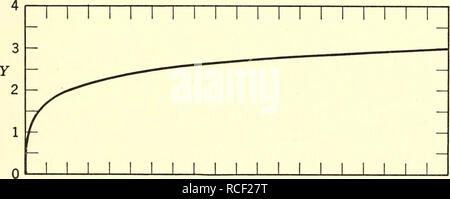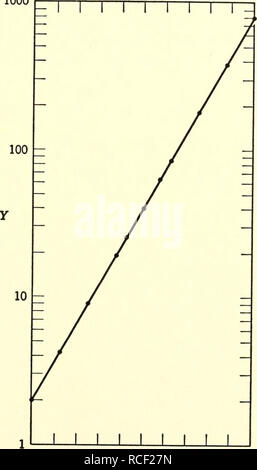RMRCF27T. Elements of statistics. Statistics. Sec. 4.5 RECTIFICATION OF THE NORMAL CURVE 103 merit of either, or both, x and y so that the new graph of ?/ = /(.r) becomes a straight line. That is, a curved line is straightened out by a change of scale. Because the reader is assumed to be familiar with logarithms, the description of a method for rectifying a normal curve will be pre- ceded by a similar discussion regarding logarithmic and exponential curves, li Y — logio X, as in Table 4.51 for selected A^'s, the pairs of values (X, Y = logio X) plot on the curve of Figure 4.51. TABLE 4.51 Some Pairs oRMRCF27N. Elements of statistics. Statistics. Sec. 4.5 RECTIFICATION OF THE NORMAL CURVE 105 logio Y — 1.30A' + 0.30, approximately. This will be a straight line if Y is measured on a logarithmic scale, as in Figure 4.54. Table 4.52 gives the values used in plotting Figure 4.54. TABLE 4.52 Values of 2e^^ for Selected X's X Y = 2e'^ X Y = 2e^^ 0 2.00 1.15 63.00 0.25 4.23 1.25 85.04 0.50 8.96 1.50 180.03 0.75 18.98 1.75 381.14 0.85 25.61 2.00 806.86 1.00 40.17 1000. 0 0.2 0.4 0.6 0.8 1.0 1.2 1.4 1.6 1.8 2.0 X Figure 4.54. Graph of F = 2e^^ on semi-log paper.. Please note that these images are extracted